Next: User Interface Up: Warping Previous: Element Pairs

## Collections of Element Pairs

In extending the warping algorithm of the previous paragraph to multiple element pairs, we adhere to the intuitive mental model of magnetic sculpting used in . Each pair of elements defines a field that extends throughout the volume. A collection of element pairs defines a collection of fields, all of which influence each point in the volume. We therefore use a weighted averaging scheme to determine the point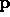inthat corresponds to each pointof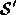. That is, we first compute to what point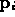each element pair would map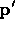in the absence of all other pairs; then, we average the's using a weighting function that depends on the distance ofto the interpolated elements.

Our weighting scheme uses an inverse square law:is weighted by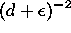where d is the distance offrom the element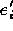;is a small constant used to avoid division by zero.The type of elementdetermines how d is calculated:

Points:
d is the distance betweenand the origin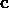of the local coordinate system of element. This definition is identical to .
Segments:
The element is treated as a line segment centered at the origin, aligned with the local x-axis and having length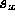; d is the distance offrom this line segment. This definition is identical to .
Rectangles:
Rectangles have the same center and x extent as segments, but also extend into a second dimension, having width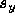along the local y-axis. d is zero ifis on the rectangle, otherwise it is the distance offrom the rectangle. This definition extends segments to area elements.
Boxes:
Boxes add depth to rectangles, thus extending for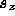units along the local z-axis. d is zero ifis within the box, otherwise it is the distance offrom the box's surface.

The reader will notice that the point, segment, and rectangle element types are redundant, as far as the mathematical formulation of our warp is concerned. However, a variety of element types maintains best the intuitive conceptual analogy to magnetic sculpting.Next: User Interface Up: Warping Previous: Element Pairs

Last update: 11 May 1995 by Apostolos "Toli" Lerios
tolis@cs.stanford.edu